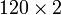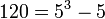# Direct product of SL(2,5) and Z2

View a complete list of particular groups (this is a very huge list!)[SHOW MORE]

## Definition

This group is defined as the external direct product of special linear group:SL(2,5) and cyclic group:Z2.

## Arithmetic functions

Want to compare and contrast arithmetic function values with other groups of the same order? Check out groups of order 240#Arithmetic functions
Function Value Similar groups Explanation
order (number of elements, equivalently, cardinality or size of underlying set) 240 groups with same order order of direct product is product of orders: the order is the product$120 \times 2$ where$120 = 5^3 - 5$ is the order of special linear group:SL(2,5) and 2 is the order of cyclic group:Z2.

## GAP implementation

### Group ID

This finite group has order 240 and has ID 94 among the groups of order 240 in GAP's SmallGroup library. For context, there are 208 groups of order 240. It can thus be defined using GAP's SmallGroup function as:

SmallGroup(240,94)

For instance, we can use the following assignment in GAP to create the group and name it$G$:

gap> G := SmallGroup(240,94);

Conversely, to check whether a given group$G$ is in fact the group we want, we can use GAP's IdGroup function:

IdGroup(G) = [240,94]

or just do:

IdGroup(G)

to have GAP output the group ID, that we can then compare to what we want.

### Other descriptions

Description Functions used
DirectProduct(SL(2,5),CyclicGroup(2)) DirectProduct, SL, CyclicGroup